Top > Technical Information > Mechanical Properties

##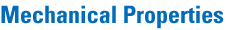### 1. Knoop Hardness* (HK)

Knoop hardness is used to characterize the hardness of the surface of optical glass against penetration.For this measurement a pyramid-shaped diamond indenter with vertex-angles 172°30' and 130°and with a rhombic base is applied to the polished specimen surface.Indentation loads of up to 0.9807N are applied for 15 seconds. The size of the resulting indentation is then measured. Knoop hardness, HK, is calculated using the following equation.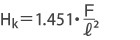Where F(N) denotes the applied load and l (mm) is the length of the longer diagonal of the resulting indentation.

 Notes 1: The Knoop hardness is expressed in terms of MPa or N/mm2, which is omitted herein accordance with common usage. Notes 2: The HK value obtained by the above equation using SI units is equal to that obtained by the calculation equation using kgf units. Notes 3: 1N = 1.01972×10-1kgf Notes 4: The Knoop hardness measurements shown in this catalog are based on the classifications in table 12 .

Table12 Classes of Knoop Hardness (HK)

Class Knoop Hardness
1 < 150
2 ≥ 150 - < 250
3 ≥ 250 - < 350
4 ≥ 350 - < 450
5 ≥ 450 - < 550
6 ≥ 550 - < 650
7 ≥ 650

### 2. Abrasion Factor* (FA)

The abrasion factor*, FA, is a relative measure for lapping. A glass specimen with a surface area of 9cm2 is placed 80mm from the center of a cast iron circular plate. The plate is then rotated horizontally at 60 r.p.m., and a 9.807N lapping weight is vertically loaded on the specimen. Lapping is continued for five minutes, with a continuous supply of lapping compound composed of 10g aluminum oxide (grain size: 20μm) in 20ml of water. The mass loss of the specimen, m, is then measured and compared to that of the standard reference material (BSC7), mo, specified by JOGIS. The abrasion factor is then determined by the following equation: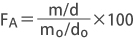where d is the specific gravity of the test specimen and do is the specific gravity of the standard reference material (BSC7). See the attached HK-FA diagram for details.

### 3. Elastic Properties (E, G, μ)

Young's modulus E and the modulus of rigidity, G, are measured by an acoustic method on a well-annealed 20×20×100(mm) specimen placed in an isothermal chamber. The velocity of both the
longitudinal and transverse waves of 5 MHz ultrasonic waves are measured. Young's modulus E and the modulus of rigidity G are then calculated by the following equations:
where Vl = velocity of the longitudinal wave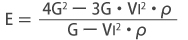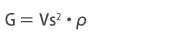ρ = density of the glass
ratio μ is obtained by the following equation: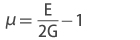Note1:
Young's modulus is termed the modulus of longitudinal elasticity. The modulus of rigidity is also termed the modulus of transverse elasticity or shear modulus.
Note2:
1GPa = 1.01972×102 kgf / mm2

It is measured by the method of applying to JIS R1602:1995.

### 4. Flexural Strength (Modulus of Rupture)(σb)

A well-annealed specimen of 4mm in width, 3mm in thickness, and 40mm in total length with polished upper and lower surfaces and a chamfered edge of C0.2 is used to measure its breaking load P(N) by the "3-point bending test" according to JIS R 1601-1981 and the flexural strength, σb, is calculated by the following equation: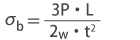where L is the support span(mm), W is the width(mm) of the specimen and t is the thickness(mm) of the specimen. The measured value is expressed in MPa.

Note.1: Mpa = 1.01972 × 10-1 kgf / mm2